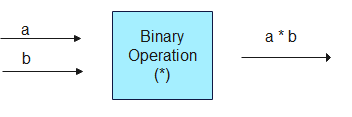# Binary Operations

## Introduction to Binary Operation

• Binary operations are mathematical operations that are performed on two operands or values. In other words, they are operations that involve two inputs or arguments. The most common binary operations are addition, subtraction, multiplication, division, and exponentiation.
• For example, in the expression 5 + 3, the plus sign (+) represents the binary operation of addition. The operands are 5 and 3, and the result of the operation is 8.
• Another example is the expression 10 / 2. The forward slash (/) represents the binary operation of division, with the operands being 10 and 2, and the result being 5.
• Binary operations are used in many areas of mathematics, including algebra, geometry, calculus, and more. They are also used extensively in computer science, where binary operations are used to manipulate and process binary data,such as numbers represented in binary code.
• In this page, we will see the general definition of binary operations in terms of functions and relations

## Definition of Binary Operations• A binary operation * on a set A is a function * : A x A -> A
• we denote * (a,b) by a * b
Example
Show that addition, multiplication are binary operations on N ( Set of Natural Numbers), but subtraction and division is not a binary operation on N.
Solution
+ : N x N -> N
It is given by
+ (a,b) = a + b
Clearly a+b belongs to set of Natural Numbers
So, it is a binary operation
- : N x N -> N
It is given by
- (a,b) = a - b
Now a-b is not necessary natural numbers as 4 -5=-1
So, it is not a binary operation
x : N x N -> N
It is given by
x (a,b) = a x b
Clearly a x b belongs to set of Natural Numbers
So, it is a binary operation
÷ : N x N -> N
It is given by
÷ (a,b) = a ÷ b
Now a ÷ b is not necessary natural numbers as 1/2 is not natural numbers
So, it is not a binary operation

## Properties of Binary Operations

Commutative Property
A binary operation ∗ on the set X is called commutative, if a ∗ b = b ∗ a, for every a, b ∈ X.
Associative Property
A binary operation ∗ : A x A -> A is said to be associative if (a ∗ b) ∗ c = a ∗ (b ∗ c), for all a, b, c, ∈ A
Identity Property
Given a binary operation ∗ : A x A -> A, an element e ∈ A, if it exists, is called identity for the operation ∗, if a ∗ e = a = e ∗ a, for all a ∈ A
Inverse Property
Given a binary operation ∗ : A x A -> A with the identity element e in A, an element a ∈ A is said to be invertible with respect to the operation ∗, if there exists an element b in A such that a ∗ b = e = b ∗ a and b is called the inverse of a and is denoted by a-1

## Solved Example

Example 1
Find out if the binary operation ∗ : R × R → R defined by a ∗ b = a + 3b
(i) Commulative
(ii) Associative
Solution
(i) For commulative
a* b =a + 3b
b* a =b + 2a
Clearly this is not commulative
(ii) For Associative
(a ∗ b) ∗ c = a ∗ (b ∗ c)
The operation ∗ is not associative, since
(8 ∗ 5) ∗ 3 = (8 + 10) ∗ 3 = (8 + 10) + 6 = 24,
while 8 ∗ (5 ∗ 3) = 8 ∗ (5 + 6) = 8 ∗ 11 = 8 + 22 = 31
Example 2
Let A= R x R and * be the binary operation on A defined by
(a,b)* ( c,d) = (a+c , b+d)
(i) Show that * is commutative and Associative
(ii) Find the identity element for * on A
(iii) Find the inverse of every element (a,b) ∈ A
Solution
(i)Commutative:
(a,b)∗(c,d)=(a+c,b+d)
(c,d)∗(a,b) =(c+a,d+b)= (a+c, b+d)
So , ∗ is commutative.
Associative:
((a,b)∗(c,d))∗(e,f)=((a+c,b+d)) ∗(e,f)
=(a+c+e,b+d+f)
(a,b)∗((c,d))∗(e,f))=(a,b)∗(c+e,d+f)
=(a+c+e,b+d+f)
Hence ∗ is associative.
(ii) Identity element:
Let (e1,e2) be the identity element
then by definition
(a+ e1) *(b + e2) = (a, b) = (e1 + a)​ *(e2 + b)
[(a+ e1),(b + e2)]=(a, b) = [(a + e)​ ,(b + e2)]
So a+ e1=a and b+e2=a
Hence e1=e2=0
So (0,0) is the identity element
(iii)Inverse:
Let (i1,i2) is inverse of element (a,b)∈ A
Now by definition.
(a+i1) * (b+i2)= 0* 0 =(i1+a) * (i2+b)
[(a+ i1),(b + i2)]=(0,0) = [(a + i1)​ ,(b + i2)]
So, a+ i1=0 and b+i2=0
So (-a,-b) is inverse of every elemnt (a,b) ∈ A.

Go back to Class 12 Main Page using below links